# Maths Brain Teasers 118 :: Can You - Complete the Pentagon?

in STEMGeekslast year

Hey All; @StemGeeks Mathematician;

Without further, ado, let's get to our puzzle for today:: Maths Brain Teasers 118 :: Can You - Complete the Pentagon?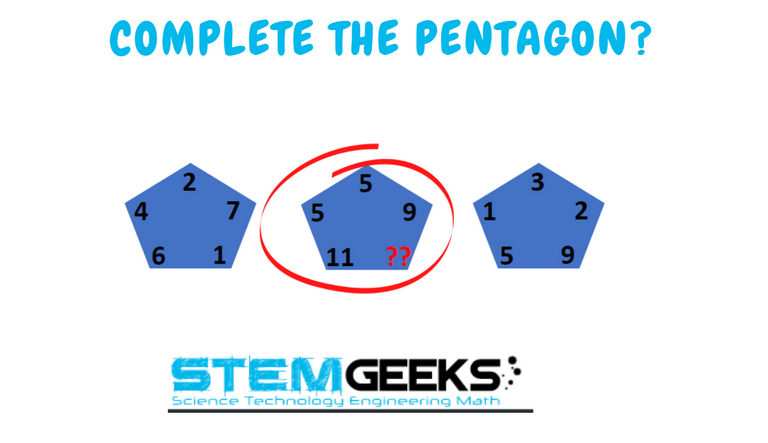Have a close look at the image given and see if you can find the correct number that replaces the question mark.

There is logic to resolve the puzzle; try finding out the logic and it should be resolved in seconds.. Guesswork isn't going to help...

With that, I'll leave you all with the Maths Brain Teasers 118 :: Can You - Complete the Pentagon? Good luck solving the puzzle...

##### Maths Brain Teasers 117 :: Can You - Complete the Rectangle?- Solved with Explanation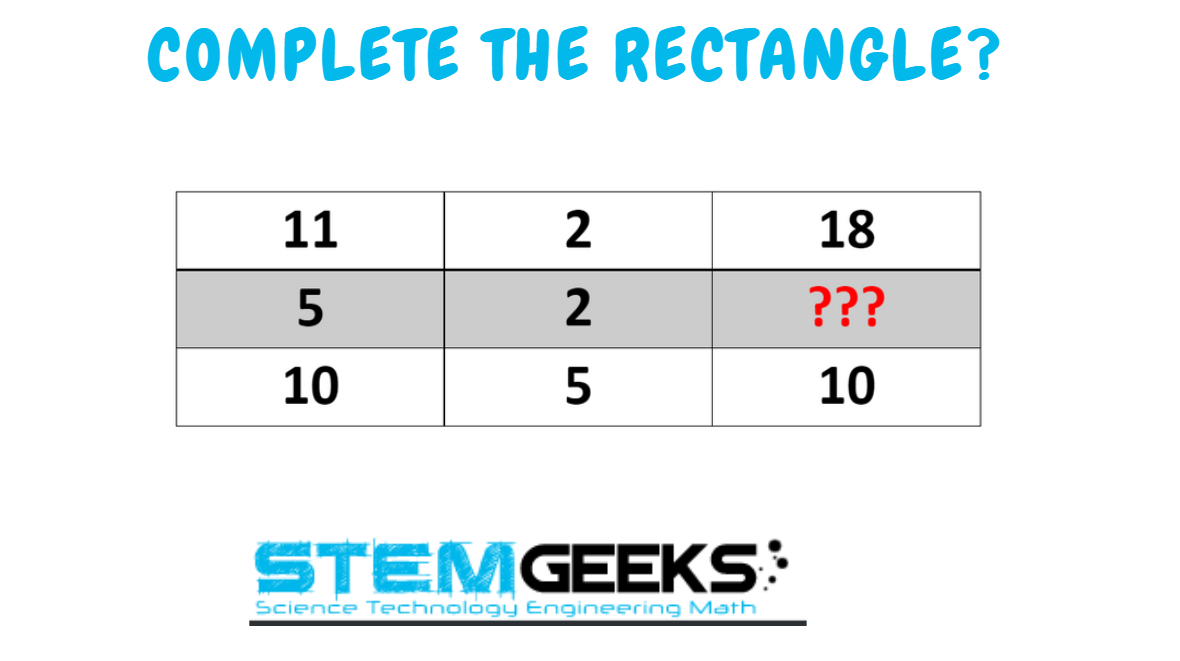Let's have a look at the Step by Step instructions to Solve the Maths Brain Teasers 116 :: Can You - ODD Letter in the CIRCLES?Maths Brain Teasers 117 :: Can You - Complete the Rectangle?

If you carefully look at the Image in question; You would have noticed the following pattern is being followed::

### Difference of first two numbers in each row and then double it to get third number in that row.

Let's take the example of the first row::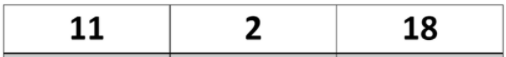### 11 - 2 = 9 * 2 = 18

In the similar fashion lets take the row in question::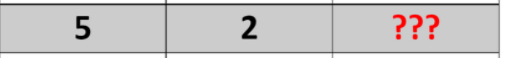### 5 - 2 = 3 * 2 = 6

Finally, the answer to the puzzle is Number:: 6

### STEM token GiveAway

I'll be again doing a giveaway of STEM tokens to the lucky random winner with the correct answer. For the last contest, which was [Maths Brain Teasers 117 :: Can You - Complete the Rectangle?]https://stemgeeks.net/hive-163521/@gungunkrishu/maths-brain-teasers-117-can-you-complete-the-rectangle).

We had 5 entries and I am happy to see the engagement. At the same time, it's great to see the detailed answers provided by everyone as to how they arrived at the solution of the puzzle.. Well done guys.. Way to GO... Now the results part; all entries provided the right answer. So I am going to use a random comment picker tool to pick one Winner. And the Winner is::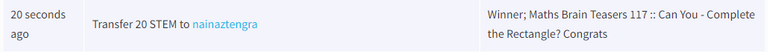### Math Quote for the Day::

Here is the motivation to solve the Maths Equation Puzzle?If you like my work, then please spread the Word.. that we do have the Math Brain Teasers competition here @StemGeeks platform. Reblog is much appreciated.

Best RegardsFind Me on the Other Social Media Platforms::PS:: All the Maths Brain Teasers; are made by me using the Pro Canva License Version

Sort:
last year (edited)

Hello the answer is 10, the formula is to subtract the number of the first pentagon from the second to get the third.
I make a photo to show it.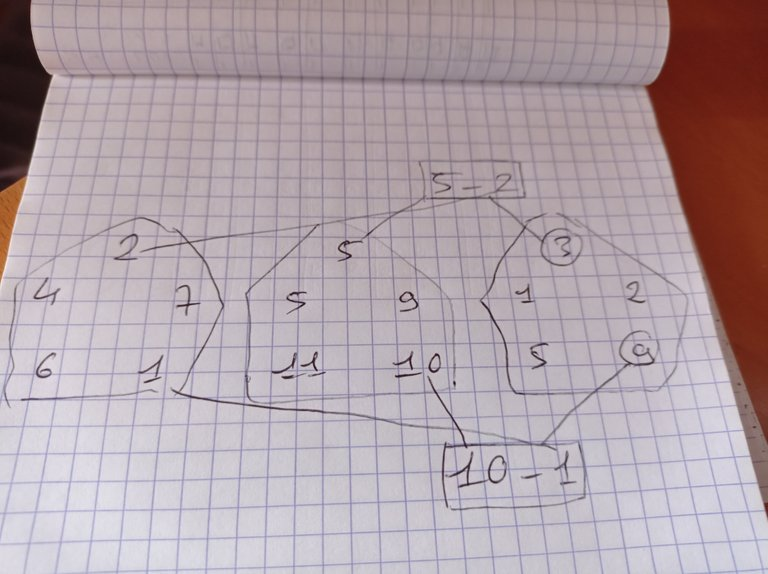last year (edited)

The missing number is 10. We add similar position of the left and right to get the middle. in case of ?? We add 9+1 =10 that's for the Pentagon.
Great quote 💪💪💪